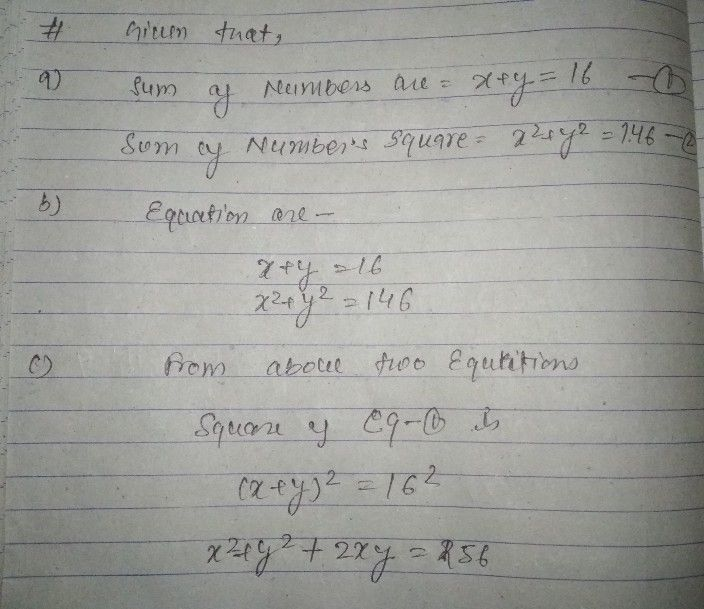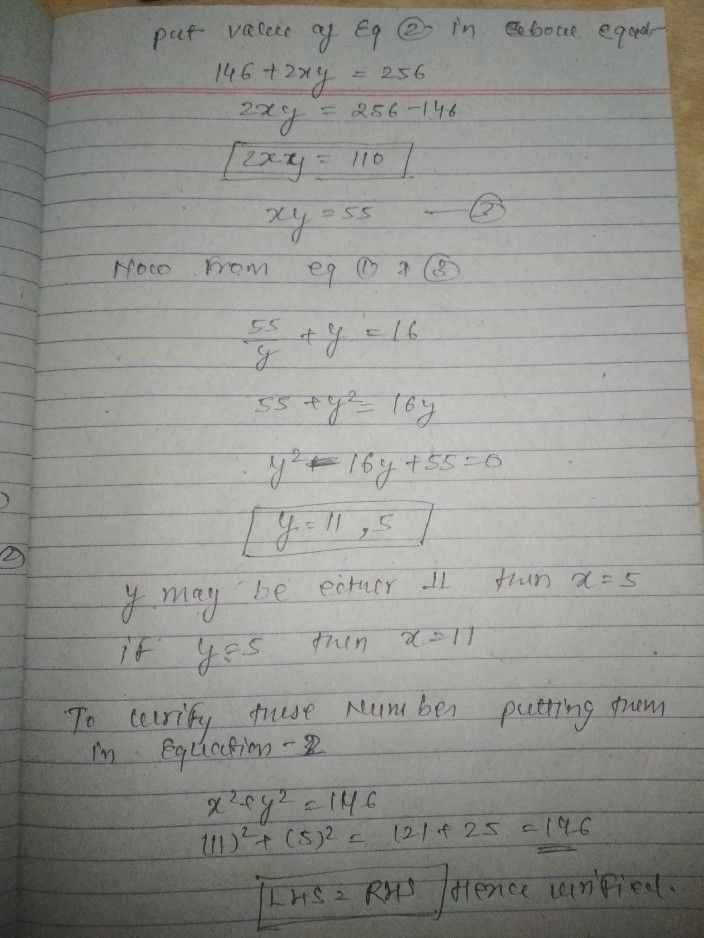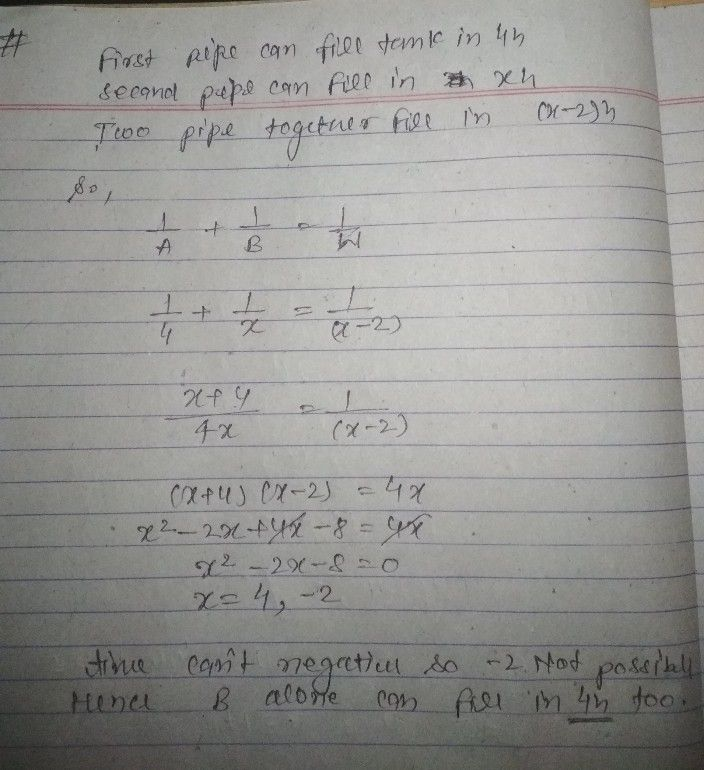Symbol
Problem$1.$ The sum $o1$ $t180$ numbers is 16, and the sum of their squares $is146$ Find the two numbers. $a$ What expressions represent the first number? How about the expressions that represent the second number? $b$ $F$ Formulate an equation relating the two numbers. Explain how you arrived at the mathematical sentence. c. Using the equation, how will you determine the first and second numbers? $c$ $d$ $c$ What is the first number? The second number? How can we sure that the two numbers are correct? Justify. $2.$ $Ty0$ pipes are used to fill $a$ water tank. The first pipe can fill a tank $in4$ $hot1rs$ alone. The two pipes can fill $a$ tank $in2$ hours less time than the second pipe alone. How long will it take the second pipe to fill the tank alone? a. How will you represent the length of the time the second pipe can fill the tank alone? How will you represent the length of the time the two pipes can fill the tank together? b. How will you represent the part of the tank filled by the first pipe alone $0$ hour? How will you represent the part of the tank filled by the sefciollned d opbiy npe e alone in one hour? How will you represent the part of the tank the two pipes together in one hour? $18$
7th-9th grade
Algebra
Search count: 111
SolutionQanda teacher - NIMKI